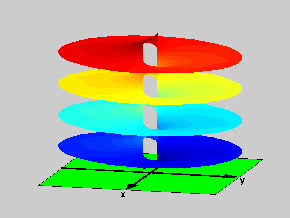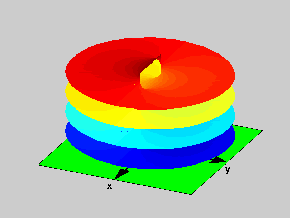18.04 | Fall 1999 | Undergraduate

# Complex Variables with Applications

Study Materials

## Riemann Surfaces: f(z)=log((z+1)/(z-1))

(All images created with MATLAB® software)

Click on pictures for more information and an image of higher resolution.Figure 1: Riemann Surface of the function f(z)=log((z+1)/(z-1))

To construct the Riemann Surface for the function f(z)=log((z+1)/(z-1)), we start with the complex plane with a single cut on the real axis (from x = -1 to x = 1), where the function has infinitely many branches. Then we proceed in the same fashion used in the previous examples. The two pictures show two views of the resulting surface.Figure 2: Top view for the Riemann Surface of the function f(z)=log((z+1)/(z-1))